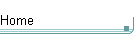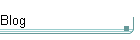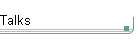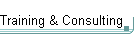GotW #30On theblogNovember 4: Other Concurrency Sessions at PDC November 3 October 26: Hoare on Testing October 23This is the original GotW problem and solution substantially as posted to Usenet. See the book Exceptional C++ (Addison-Wesley, 2000) for the most current solutions to GotW issues #1-30. The solutions in the book have been revised and expanded since their initial appearance in GotW. The book versions also incorporate corrections, new material, and conformance to the final ANSI/ISO C++ standard.

## Name LookupDifficulty: 9.5 / 10

When you call a function, which function do you call? The answer is determined by name lookup, but you're almost certain to find some of the details surprising.### Problem

In the following code, which functions are called? Why? Analyze the implications.

```    namespace A {
struct X;
struct Y;
void f( int );
void g( X );
}

namespace B {
void f( int i ) {
f( i );   // which f()?
}
void g( A::X x ) {
g( x );   // which g()?
}
void h( A::Y y ) {
h( y );   // which h()?
}
}```### Solution

In the following code, which functions are called? Why?

Two of the three cases are (fairly) obvious, but the third requires a good knowledge of C++'s name lookup rules -- in particular, Koenig lookup.

```    namespace A {
struct X;
struct Y;
void f( int );
void g( X );
}

namespace B {
void f( int i ) {
f( i );   // which f()?
}```

This f() calls itself, with infinite recursion.

There is another function with signature f(int), namely the one in namespace A. If B had written "using namespace A;" or "using A::f;", then A::f(int) would have been visible as a candidate when looking up f(int), and the "f(i);" call would have been ambiguous between A::f(int) and B::f(int). Since B did not bring A::f(int) into scope, however, only B::f(int) is considered and so the call is unambiguous.

```        void g( A::X x ) {
g( x );   // which g()?
}```

This call is ambiguous between A::g(X) and B::g(X). The programmer must explicitly qualify the call with the appropriate namespace name to get the g() he wants.

You may at first wonder why this should be so. After all, as with f(), B hasn't written "using" anywhere to bring A::g(X) into its scope, and so you'd think that only B::g(X) would be visible, right? Well, this would be true but for an extra rule that C++ uses when looking up names:

Koenig Lookup (simplified)
If you supply a function argument of class type (here x, of type A::X), then to find the function name the compiler considers matching names in the namespace (here A) containing the argument's type.

There's a little more to it, but that's essentially it. Here's an example, right out of the standard:

```    namespace NS {
class T { };
void f(T);
}

NS::T parm;
int main() {
f(parm);    // OK, calls NS::f
}```

I won't delve here into the reasons why Koenig lookup is a Good Thing (if you want to stretch your imagination, replace "NS" with "std", "T" with "string", and "f" with "operator<<" and consider the ramifications). See the "further reading" at the end for much more on Koenig lookup and its implications for namespace isolation and analyzing class dependencies. Suffice it to say that Koenig lookup is indeed a Good Thing, and that you should be aware of how it works because it can sometimes affect the code you write.

```        void h( A::Y y ) {
h( y );   // which h()?
}
}```

There is no other h( A::Y ), and so this h() calls itself with infinite recursion.

Although B::h()'s signature mentions a type found in namespace A, this doesn't affect name lookup because there are no functions in A matching the name h(A::Y).

Analyze the implications.

In short, the meaning of code in namespace B is being affected by a function declared in the completely separate namespace A, even though B has done nothing but simply mention a type found in A and there's nary a "using" in sight!

What this means is that namespaces aren't quite as independent as people originally thought. Don't start decrying namespaces just yet, though; namespaces are still pretty independent and they fit their intended uses to a T. The purpose of this GotW is just to point out one of the (rare) cases where namespaces aren't quite hermetically sealed... and, in fact, where they should _not_ be hermetically sealed, as the "further reading" shows.